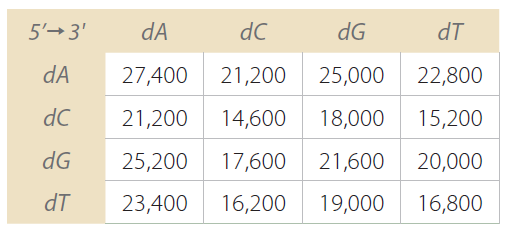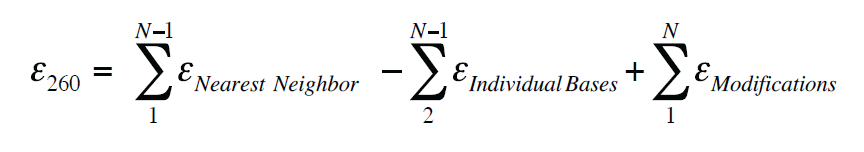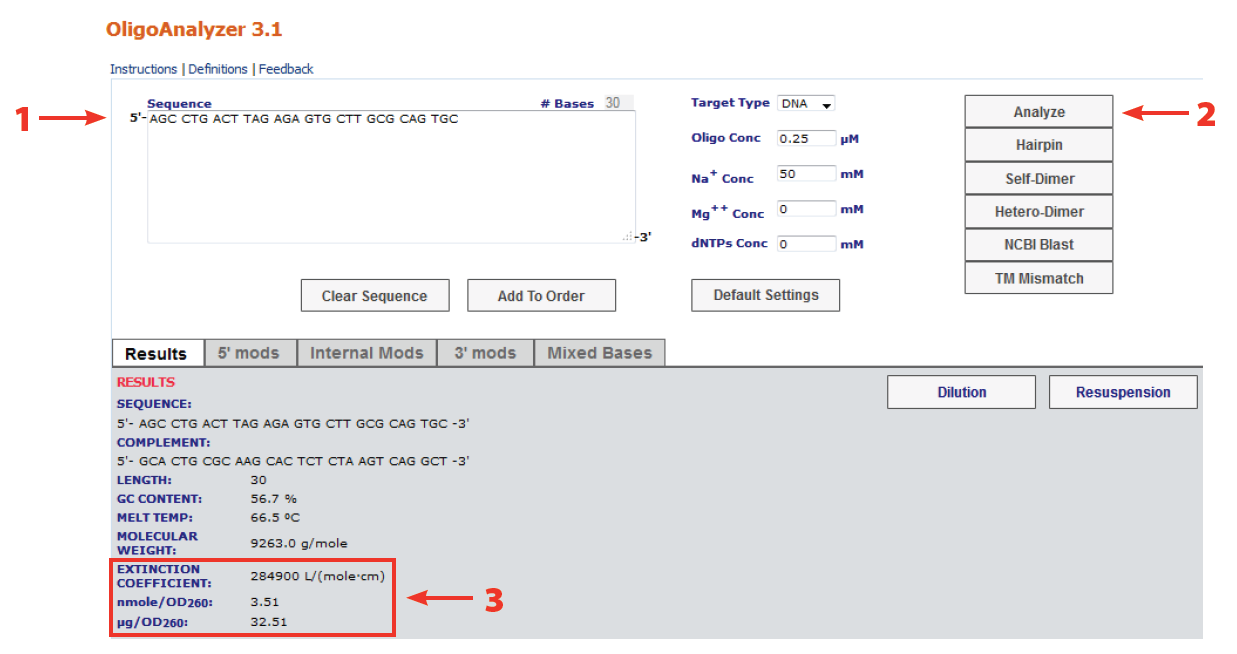# Oligo quantification—Getting it rightDid you know that a supplier's yield readings can differ from what the researcher calculates after resuspension? Learn the importance of using the right molar extinction coefficient in calculations of oligonucleotide concentration.

We occasionally get calls from researchers who take issue with the yield readings we supply, saying they differ from what the researcher has calculated after resuspension. This article highlights the importance of using the right molar extinction coefficient in calculations of oligonucleotide concentration.

## Why include extinction coefficient in calculations?

Optical absorbance at 260 nm is routinely used to measure the concentration of nucleic acids present in a solution. Approximate conversion factors estimate that duplex DNA is about 50 μg/OD260, single-stranded RNA is approximately 40 μg/OD260, and single-stranded DNA is approximately 33 μg/OD260.

While these conversion factors may provide a reasonable estimate for long, essentially randomized sequences, they are less reliable for short oligonucleotides and repeating sequences. Conjugated double bonds are strong absorbers of light. They exhibit a property known as resonance, which causes them to absorb light with longer wavelengths (i.e., low energy). The molar absorptivity or extinction coefficient, and wavelength of maximum absorbance generally increase with increasing numbers of conjugated double bonds. Thus, the absorbance of each base is different, and base composition, sequence context, and sequence length all influence absorbance. In general terms, the extinction coefficient of a material describes the amount of light absorbed at a given concentration and distance travelled.

## How much does sequence composition affect extinction coefficient?

Would you believe that the extinction coefficient for a sequence as short as 6 bases can vary just by arranging the bases in a different order? Additionally, base composition can lead to significant differences in the extinction coefficient. See Figure 1 for examples.

Therefore, rather than using estimates, it is better to calculate the exact extinction coefficient (ε260) value for each oligo. Further, calculations should take into account the presence of oligo modifications, such as fluorescent dyes, which may have significant absorbance at 260 nm.

For these reasons, IDT calculates the extinction coefficient for every oligo synthesized using a nearest neighbor method. This value is then used to measure the yield for each oligonucleotide produced.

## The nearest neighbor method

Nearest neighbor values for ε260 of dNTPs are displayed in Figure 1.We then use the formula shown in Figure 2 [2,3].

Absorbance is calculated using the Beer-Lambert Law:

A = log(Io/I) = ε × c × p

where A is absorbance, Io and I are, respectively, the intensities of incident and transmitted light, c is the molar concentration of an oligonucleotide (mole/L), p is the length of the light path through the sample (cm), and ε is the molecule molar extinction coefficient (L/mole•cm).

## Does IDT have a DNA extinction coefficient calculator?

The coefficient of extinction for any oligonucleotide sequence can be calculated using IDT's free OligoAnalyzer™ Tool which will also determine OD260 for that sequence (Figure 3).## Additional recommendations for determining the concentration of an oligo in solution based on A260 nm measurements and use of the extinction coefficient in your calculations

Know the limitations of your instrumentation. Most labs use basic spectrophotometers that are focused on accessibility and ease of use. These advantages are important but sometimes come at the expense of measurement accuracy. Know the linear detection range of your instrument and stay within that range. Additionally, like all pieces of equipment, spectrophotometers may age or drift so it is important to monitor their performance over time. All IDT spectrophotometers are regularly calibrated by IDT's Metrology department.

Thoroughly mix samples before testing. To follow best practices when assessing quantity, it is essential that the material tested is representative of the entire sample. Be sure to mix the sample to homogeneity to avoid under or over estimations of quantity. This is important not only during resuspension, but before all critical absorbance measurements. Concentration gradients may develop due to freeze-thaw cycles, for example.

Be mindful of volume delivery during sample preparation. While sample volume accuracy may not be critical for all instruments, it is very important for cuvette-based spectrophotometers. Follow best practices during sample transfer by using proper pipetting techniques and calibrated pipettes. Generally speaking, it is best to use the greatest transfer volume possible to avoid potential pipetting errors .

Published Jan 18, 2013
Revised/updated Apr 26, 2023